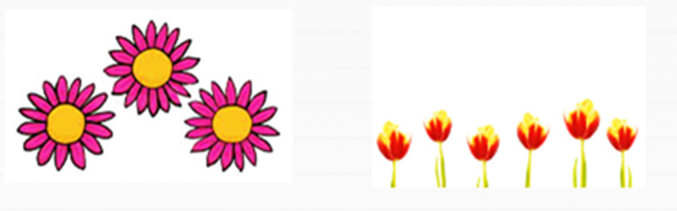Mathematics
Easy

Question

# 3 pink flowers and 6 orange flowers. How many in all?## 89107Hint:

## The correct answer is: 9

### Here, we have to find the total number of flowers.Number of pink flowers = 3 .Number of orange flowers = 6 .Therefore, total number of flowers = 3 + 6= 9 .Hence, the correct option is (b).

Add the digits of the same place value of all numbers and write the resultant.

### Related Questions to study#### With Turito Foundation.#### Get an Expert Advice From Turito.